Homework Help Question & Answers

# Use the function to find the image of v and the preimage of w. TV1, V2,...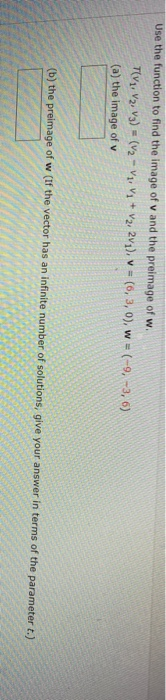Use the function to find the image of v and the preimage of w. TV1, V2, V3) = (V2 - Vz, V; + V2, 2v1), v = (6,3,0), w = (-9, -3, 6) (a) the image of v (b) the preimage of w (If the vector has an infinite number of solutions, give your answer in terms of the parameter t.)

#### Homework Answers

Answer #1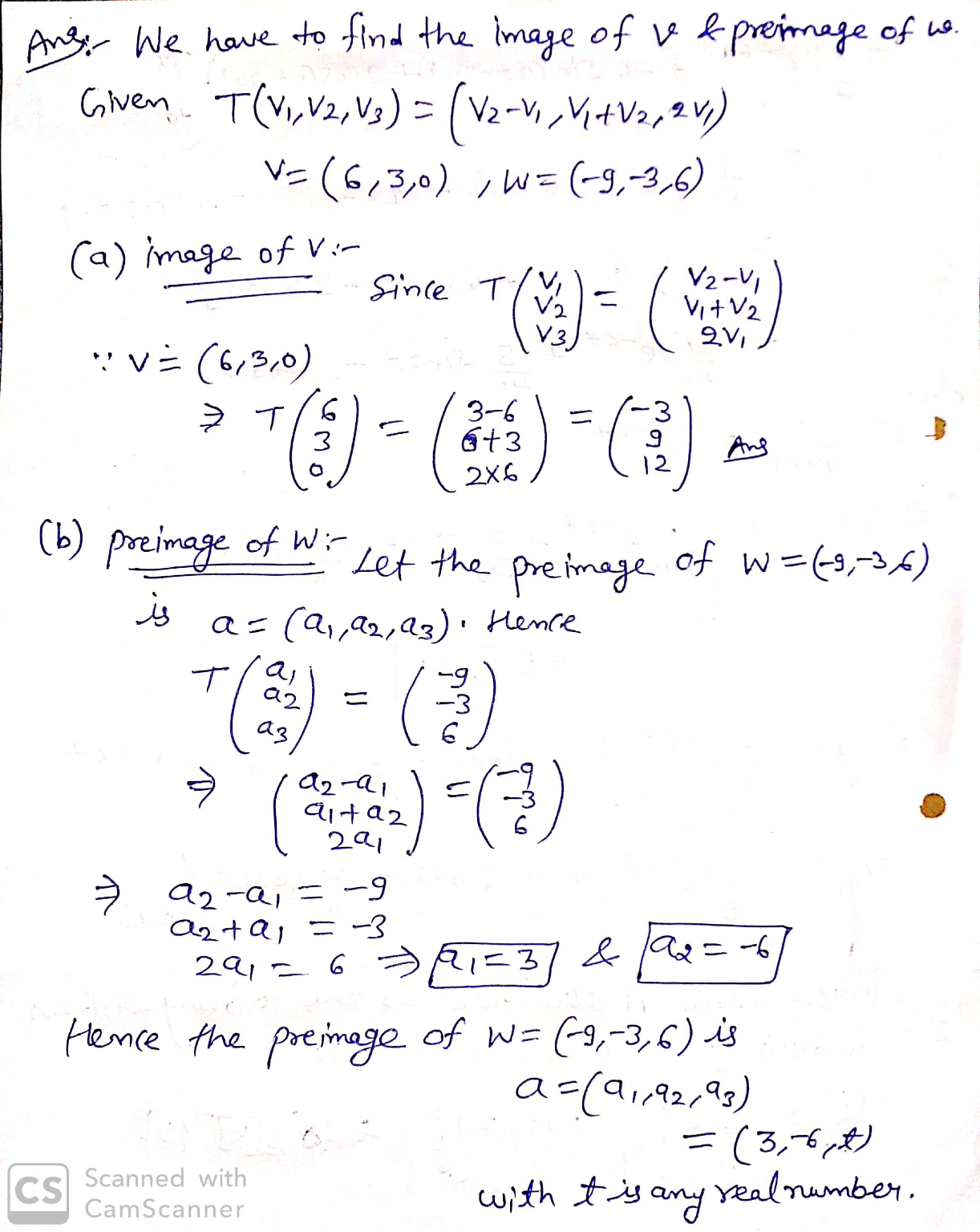Know the answer?
Your Answer:

#### Post as a guest

Your Name:

What's your source?

#### Earn Coin

Coins can be redeemed for fabulous gifts.

Not the answer you're looking for? Ask your own homework help question. Our experts will answer your question WITHIN MINUTES for Free.
Similar Homework Help Questions
• ### Use the function to find the image of v and the preimage of w. T(V1, V2,...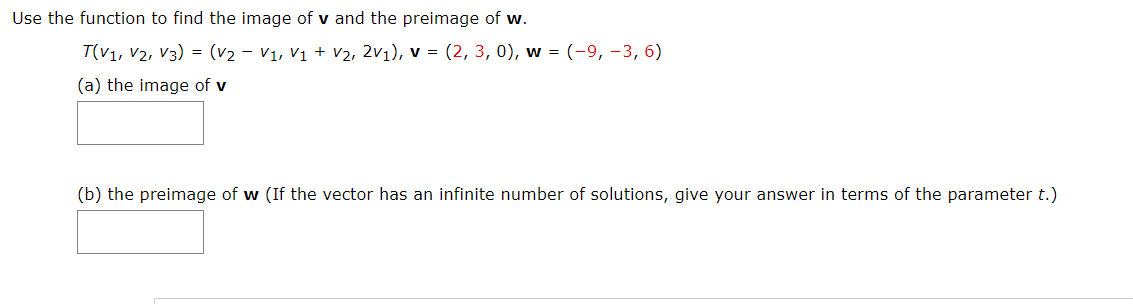Use the function to find the image of v and the preimage of w. T(V1, V2, V3) = (v2 - V1, V1 + V2, 2v1), v = (2,3,0), w = (-9, -3, 6) (a) the image of v (b) the preimage of w (If the vector has an infinite number of solutions, give your answer in terms of the parameter t.) Use the function to find the image of v and the preimage of w. TIV3, va) = (v2v2 -...

• ### Use the function to find the image of V and the preimage of W. T(V1, V2,...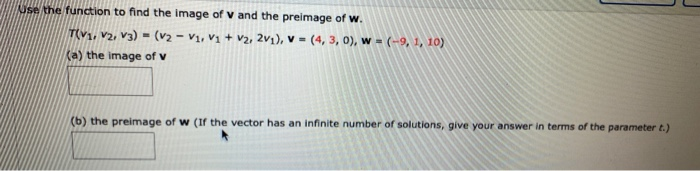Use the function to find the image of V and the preimage of W. T(V1, V2, V3) = (v2 - V1, V1 + V2, 2v1), V = (4,3,0), w = (-9, 1, 10) (a) the image of v (b) the preimage of w (If the vector has an infinite number of solutions, give your answer in terms of the parameter 1.)

• ### linear algebra Use the function to find the image of v and the preimage of w....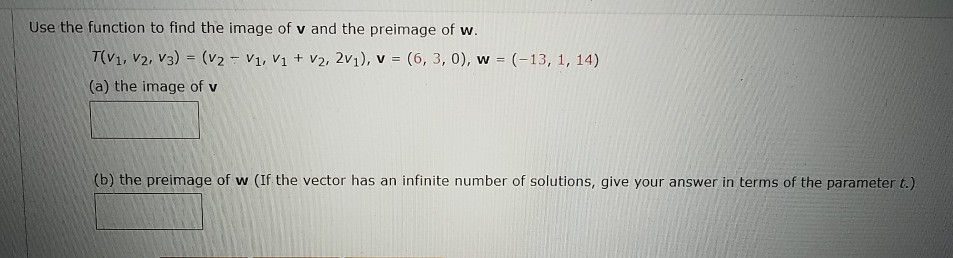linear algebra Use the function to find the image of v and the preimage of w. T(V1, V2, V3) = (v2 - V1, V1 + V2, 2v1), v = (6,3,0), w = (-13, 1, 14) (a) the image of v (b) the preimage of w (If the vector has an infinite number of solutions, give your answer in terms of the parameter t.)

• ### -/2 POINTS LARLINALG8 6.1.001. Use the function to find the image of v and the preimage...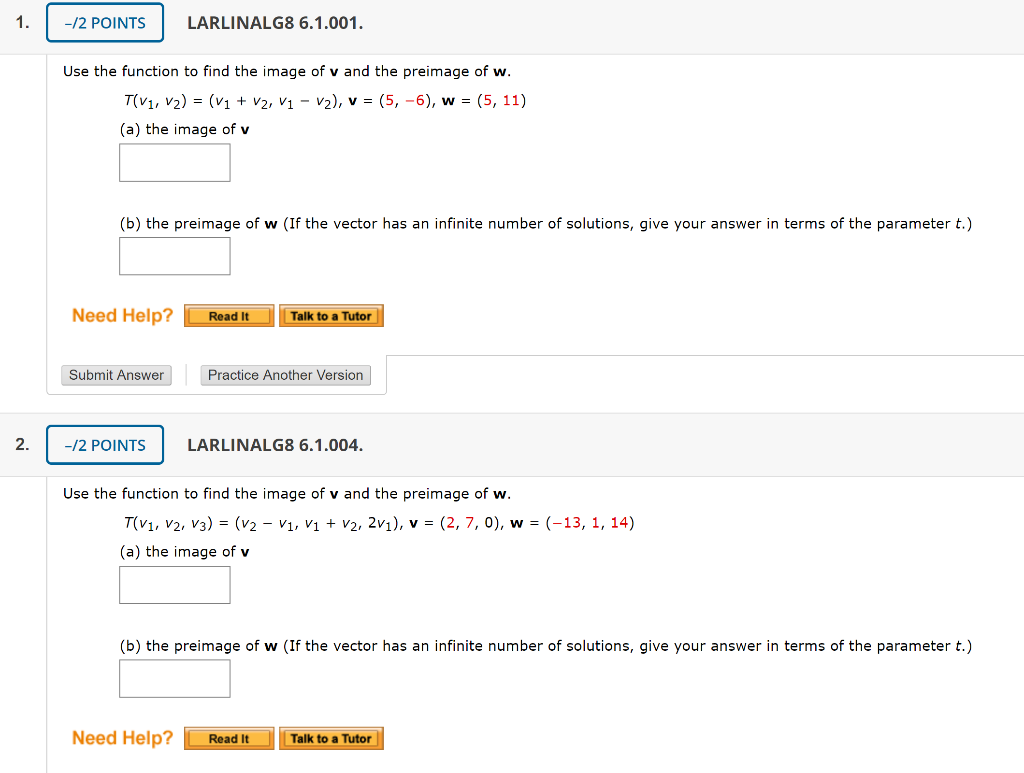-/2 POINTS LARLINALG8 6.1.001. Use the function to find the image of v and the preimage of w. T(V1, V2) = (v1 + V2, V1 - v2), v = (5, -6), w = (5, 11) (a) the image of v (b) the preimage of w (If the vector has an infinite number of solutions, give your answer in terms of the parameter t.) Need Help? Read It Talk to a Tutor Submit Answer Practice Another Version -/2 POINTS LARLINALG8 6.1.004....

• ### 0.5/1 POINTS PREVIOUS ANSWERS LARLINALG8 6.1.001. MY NOTES ASK YOUR TEACHER Use the function to find...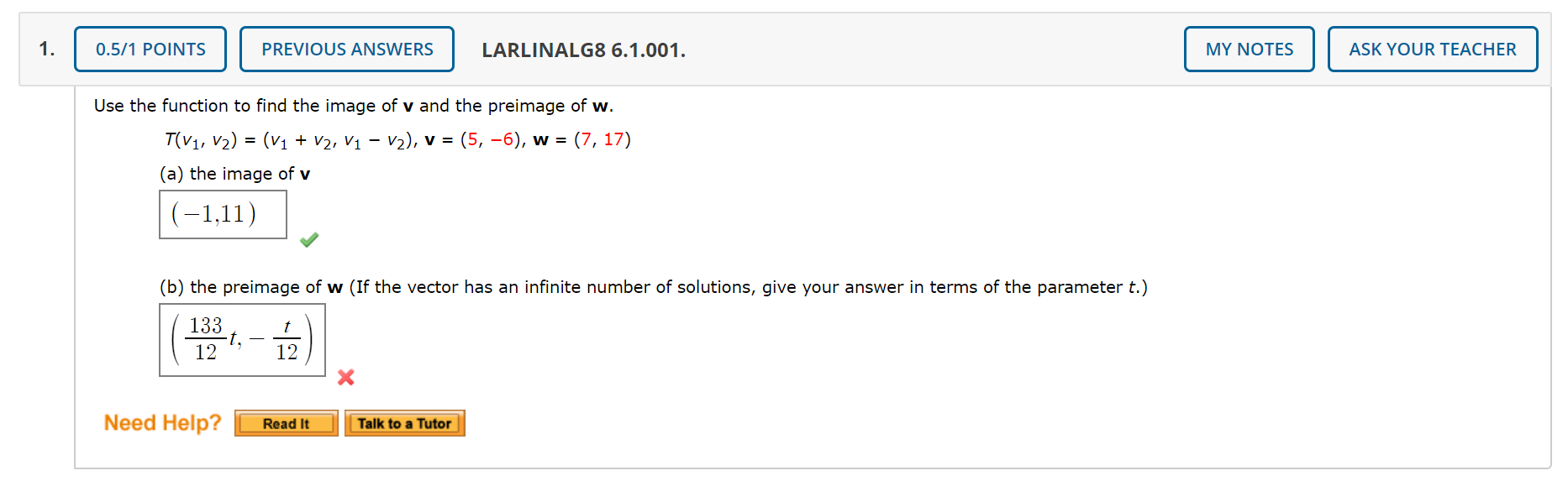0.5/1 POINTS PREVIOUS ANSWERS LARLINALG8 6.1.001. MY NOTES ASK YOUR TEACHER Use the function to find the image of v and the preimage of w. T(V1, V2) = (V1 + V2, V1 – V2), v = (5, -6), w = (7, 17) (a) the image of v |(-1,11) (b) the preimage of w (If the vector has an infinite number of solutions, give your answer in terms of the parameter t.) | 133 ( 1321,- Need Help? Read It Talk...

• ### Linear Aljebra Let B = {vy, V2, V3) be a basis for R in which we...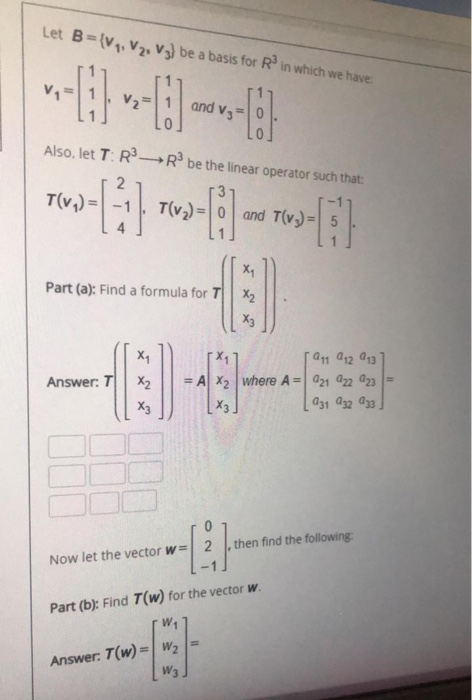Linear Aljebra Let B = {vy, V2, V3) be a basis for R in which we have and V3 Also, let TR-R be the linear operator such that: T(v.) = T(v2) and T(v.) = -0 X1 Part (a): Find a formula for T X₂ X, Answer: T X2 -0 [Ogg 912 943 = A x2 where A = 421 422 423 х3 231 232 233 Xz 0 } then find the following: Now let the vector w= Part (b): Find...

• ### Moodle Let B={V, V2, Vz) be a basis for Rin which we have: Question 2 Not...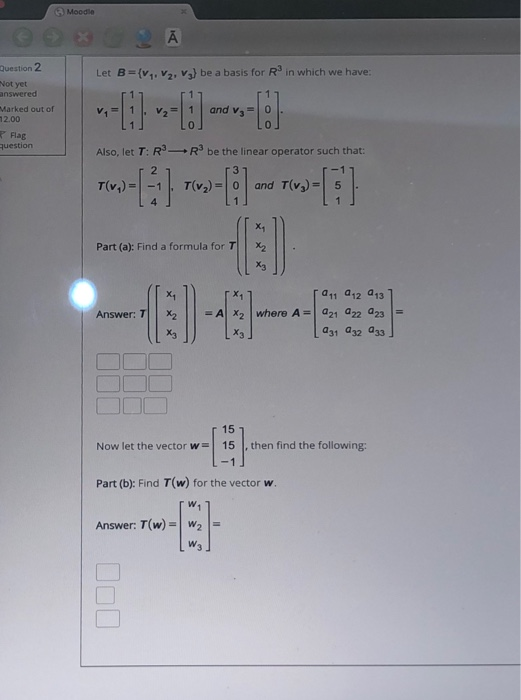Moodle Let B={V, V2, Vz) be a basis for Rin which we have: Question 2 Not yet answered Marked out of 12.00 V1 and vg = 0 - Flag question Also, let TR-R be the linear operator such that: 3 T(v.) T(v2) and T(v2) = X1 Part (a): Find a formula for T X2 X3 X Answer: 7 X2 -0 ag, 912 913 X2 where A=221 22 23 = ха 031 32 33 X2 15 Now let the vector w=...

• ### 1) Determine if w is in the subspace spanned by v1, v2, v3 2) Are the...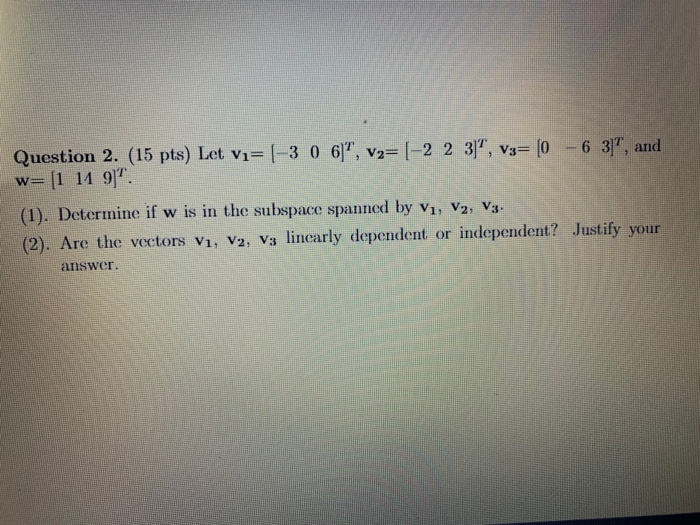1) Determine if w is in the subspace spanned by v1, v2, v3 2) Are the vectors v1, v2, v3 linearly dependent or independent? justify your answer Question 2. (15 pts) Let vi=(-3 0 6)", v2= (-2 2 3]", V3= (0 - 6 37, and w= [1 11 9". (1). Determine if w is in the subspace spanned by V1, V2, V3. (2). Are the vectors V1, V2, V3 linearly dependent or independent? Justify your answer

• ### DETAILS 14. [-/2 Points! LARLINALG8 6.1.043. MY NOTES ASK YOUR TEACHER PRACTICE ANOTHER For the linear...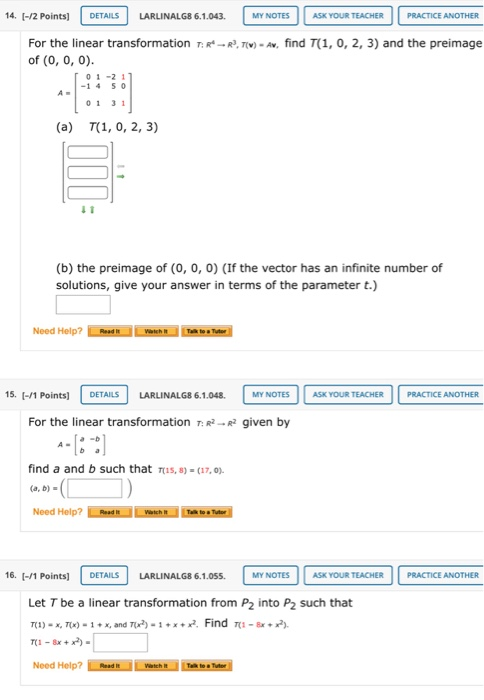DETAILS 14. [-/2 Points! LARLINALG8 6.1.043. MY NOTES ASK YOUR TEACHER PRACTICE ANOTHER For the linear transformation TR --R, TV) - Av, find (1, 0, 2, 3) and the preimage of(0, 0, 0). 01-21 -1 4 50 01 31 (a) T(1, 0, 2, 3) 11 (b) the preimage of (0, 0, 0) (If the vector has an infinite number of solutions, give your answer in terms of the parameter t.) Need Help? Rode DETAILS MY NOTES ASK YOUR TEACHER PRACTICE...

• ### Let {v1, v2,v3} be a linearly independent set in R^n and let v = -αv3 +v1,w...

Let {v1, v2,v3} be a linearly independent set in R^n and let v = -αv3 +v1,w = v2 - αv1, u= v3-αv2 where αER, find all the values of α, where v, w, u are linearly dependent. do not use matrices.